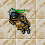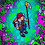## Wednesday, March 15, 2023

1.Moving the last arrow to first position makes it:
IX x IV = XXXVI
9 x 4 = 36

2.IX x IV = XXXVI
Sir coor

3.X x IV = XXXVII
IX x IV = XXXVI

Nick: The Heringer

4.The equation becomes correct by moving the I from the end to the beginning.
IX x IV = XXXVI

5.The equation becomes correct by moving the I from the end to the beginning.

IX x IV = XXXVI

- Essiee

6.Move last arrow and place it in front of the first X and the result will be ( IX x IV = XXXVI ) “9x4 = 36” Character Name: Hymura

7.move the last arrow to the beginning of the equation, thus getting 9x4=36
bomdrax (yonabra)

8.IX × IV = XXXVI

Eveh

9.Move the last arrow to be in front of the first X. The equation will become IX (9) x IV (4) = XXXVI (36) Character- Libi

10.IX × IV = XXXVI
- Hunter of Dragoes

11.Character: Arcai
Move the last arrow to be the first so it reads 9x4=36.

12.Moving last arrow (from the result) to the start of the equation obtain:
IX * IV = XXXVI
Wrong: 10 * 4 = 37
Right: 9 * 4 = 36

Character Name: Dyaniixz

13.Moving last arrow (from the result) to the start of the equation obtain:
IX * IV = XXXVI
Wrong: 10 * 4 = 37
Right: 9 * 4 = 36

Character Name: Dyaniixz

14.IX * IV = XXXVI

15.9x4=36

16.Tamarizz - Solidera

The LAST arrow to the beginning, making the X a IX
so it would be: ( IX ) ( IV ) = XXXVI

9x4=36

17.IX * IV = XXXVI

18.IX x IV = XXXVI

19.Psykeeh

IX x IV = XXXVI

20.Lyh

https://imgur.com/a/NiHLZTD

21.Last arrow to front of line, 9x4=36

22.Meregirl

https://imgur.com/a/nhTpmkk

23.IX × IV = XXXVI

24.x4Character: Mikhelle
Answer: Move the last arrow to the first place to the left (before X) and will get: IX x IV = XXXVI or 9x4=36

25.Daniellee
IX x IV = XXXVI

26.IX x IV = XXXVI
Xavlar

27.IX x IV = XXXVI
Shizurie

28.IX x IV = XXXVI

29.Move the last I in front of the first X to create 9x4=36 or IX x IV = XXXVI
Character Nep Froggod

30.PAN CETA

IX x IV = XXXVI

31.IX x IV = XXXVI

Righo

32.ix x iv = xxxvi 9 x 4 = 36

Lervan

33.IX x IV = XXXVI

Righo

34.Character: Tiger Backwoods
Move the last arrow to the first spot in the equation to make it:
IX * IV = XXXVI
9 * 4 = 36

35.Name Char Hinata: Hyuga Byakugan

Respuesta: IX x IV = XXXVI

36.Name Char: Kathryna

Respuesta: IX x IV = XXXVI

37.Respuesta: IX x IV = XXXVI

38.Name Char: Yauraill Maleld

Respuesta: IX x IV = XXXVI

39.Name Char: Thardorine Zhan
Respuesta: IX x IV = XXXVI

40.Name:Chaviitoh
Respuesta: IX x IV = XXXVI

41.Name Chart: Kriszaks
Respuesta: IX x IV = XXXVI

42.Name Chart: Alhejandra
Respuesta: IX x IV = XXXVI

43.IX x IV = XXXVI
Babygirrl

44.IX x IV = XXXVI

Green Khepri

45.IX * IV = XXXVI
-Soma deux

46.Name chart: Neutrozx
Respuesta: IX x IV = XXXVI

47.We have to pass from X x IV = XXXVII (10 x 4 = 37) to IX x IV = XXXVI (9 x 4 = 36) so it result 36 = 36
-Sapo Dungeon

48.Just need to move one arrow from right to the first X and that's (should end like IX x IV = XXXVI or 36 = 36)
-Tanabata

49.We need to move an arrow from the right for make IX x IV = XXXVI (which result as 36 = 36)
-Bara de Pan

50.Move the last arrow to the right and place it to the left before the X and the result Will be IX x IV = XXXVI / 9x4 = 36

Ramona Toledo

51.You have to move the arrow that its located all the way to the right and put it to the left before the X
IX x IV = XXXVI

Sky Elie <3

52.Presckot

moving the last arrow to the first place of the image, before the X

53.Kriizs

[img]https://i.imgur.com/XD7Yn8w.png[/img]

54.https://imgur.com/4gpVQI2

Glexzs - Lobera

55.Chart name: Korynita
Respuesta: la ecuación correcta es IX x IV = XXXVI

56.9 x 4 = 36
Samuray de carro :)
mueve la última arrow y colócala detrás del primer número :p
l X * lV = X X X V l

57.9 x 4 = 36
Samuray de carro :)
mueve la última arrow y colócala detrás del primer número :p
l X * lV = X X X V l

58.IX x IV = XXXVI

Amy Meow

59.Hi!
Answer is: IX × IV = XXXV
Cinderella Sea

60.IX x IV = XXXVI 9x4=36

Bea Charlover aca